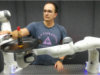### Abstract

Probability distributions are key components of many learning from demonstration (LfD) approaches. While the configuration of a manipulator is defined by its joint angles, poses are often best explained within several task spaces. In many approaches, distributions within relevant task spaces are learned independently and only combined at the control level. This simplification implies several problems that are addressed in this work. We show that the fusion of models in different task spaces can be expressed as a product of experts (PoE), where the probabilities of the models are multiplied and renormalized so that it becomes a proper distribution of joint angles. Multiple experiments are presented to show that learning the different models jointly in the PoE framework significantly improves the quality of the model. The proposed approach particularly stands out when the robot has to learn competitive or hierarchical objectives. Training the model jointly usually relies on contrastive divergence, which requires costly approximations that can affect performance. We propose an alternative strategy using variational inference and mixture model approximations. In particular, we show that the proposed approach can be extended to PoE with a nullspace structure (PoENS), where the model is able to recover tasks that are masked by the resolution of higher-level objectives.

### Bibtex reference

@article{Pignat22IJRR,
author="Pignat, E. and Silv\'erio, J. and Calinon, S.",
title="Learning from Demonstration using Products of Experts: Applications to Manipulation and Task Prioritization",
journal="International Journal of Robotics Research ({IJRR})",
year="2022",
volume="41",
number="2",
pages="163--188",
doi="10.1177/02783649211040561"
}